International Tables for Crystallography (2006). Vol. F, ch. 18.5, pp. 403-418   | 1 | 2 | https://doi.org/10.1107/97809553602060000697

Contents

• 18.5. Coordinate uncertainty  (pp. 403-418)
• 18.5.1. Introduction  (p. 403) | html | pdf |
• 18.5.1.1. Background  (p. 403) | html | pdf |
• 18.5.1.2. Accuracy and precision  (p. 403) | html | pdf |
• 18.5.1.3. Effect of atomic displacement parameters (or `temperature factors')  (p. 403) | html | pdf |
• 18.5.2. The least-squares method  (pp. 404-405) | html | pdf |
• 18.5.2.1. The normal equations  (p. 404) | html | pdf |
• 18.5.2.2. Weights  (p. 404) | html | pdf |
• 18.5.2.3. Statistical descriptors and goodness of fit  (pp. 404-405) | html | pdf |
• 18.5.3. Restrained refinement  (pp. 405-406) | html | pdf |
• 18.5.3.1. Residual function  (p. 405) | html | pdf |
• 18.5.3.2. A very simple protein model  (pp. 405-406) | html | pdf |
• 18.5.3.3. Relative weighting of diffraction and restraint terms  (p. 406) | html | pdf |
• 18.5.4. Two examples of full-matrix inversion  (pp. 406-409) | html | pdf |
• 18.5.4.1. Unrestrained and restrained inversions for concanavalin A  (pp. 406-408) | html | pdf |
• 18.5.4.2. Unrestrained inversion for an immunoglobulin  (p. 408) | html | pdf |
• 18.5.4.3. Comments on restrained refinement  (p. 408) | html | pdf |
• 18.5.4.4. Full-matrix estimates of precision  (pp. 408-409) | html | pdf |
• 18.5.5. Approximate methods  (p. 409) | html | pdf |
• 18.5.5.1. Block calculations  (p. 409) | html | pdf |
• 18.5.5.2. The modified Fourier method  (p. 409) | html | pdf |
• 18.5.5.3. Application of the modified Fourier method  (p. 409) | html | pdf |
• 18.5.6. The diffraction-component precision index  (p. 410) | html | pdf |
• 18.5.6.1. Statistical expectation of error dependence  (p. 410) | html | pdf |
• 18.5.6.2. A simple error formula  (p. 410) | html | pdf |
• 18.5.6.3. Extension for low-resolution structures and use of R free   (p. 410) | html | pdf |
• 18.5.6.4. Position error  (p. 410) | html | pdf |
• 18.5.7. Examples of the diffraction-component precision index  (pp. 411-412) | html | pdf |
• 18.5.7.1. Full-matrix comparison with the diffraction-component precision index  (p. 411) | html | pdf |
• 18.5.7.2. Further examples of the DPI using R   (p. 411) | html | pdf |
• 18.5.7.3. Examples of the DPI using R free   (pp. 411-412) | html | pdf |
• 18.5.7.4. Comments on the diffraction-component precision index  (p. 412) | html | pdf |
• 18.5.8. Luzzati plots  (pp. 412-414) | html | pdf |
• 18.5.8.1. Luzzati's theory  (pp. 412-413) | html | pdf |
• 18.5.8.2. Statistical reinterpretation of Luzzati plots  (pp. 413-414) | html | pdf |
• 18.5.8.3. Comments on Luzzati plots  (p. 414) | html | pdf |
• References | html | pdf |
• Figures
• Fig. 18.5.4.1. Plots of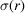versusfor concanavalin A with 0.94 Å data, ( a ) restrained full-matrix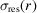, ( b ) unrestrained full-matrix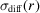(p. 407) | html | pdf |
• Fig. 18.5.4.2. Plots of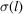versus averagefor concanavalin A with 0.94 Å data, ( a ) restrained full-matrix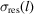, ( b ) unrestrained full-matrix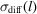(p. 407) | html | pdf |
• Fig. 18.5.4.3. Plot ofversusfrom an unrestrained full matrix for immunoglobulin mutant (T39K) with 1.70 Å data  (p. 408) | html | pdf |
• Fig. 18.5.4.4. Plot ofversus averagefrom an unrestrained full matrix for immunoglobulin mutant (T39K) with 1.70 Å data  (p. 408) | html | pdf |
• Fig. 18.5.8.1. Luzzati plots showing the refined R factor as a function of resolution for 1TGI (solid squares) and 1TGF (open squares) (Daopin et al ., 1994)  (p. 413) | html | pdf |
• Tables
• Table 18.5.7.1. Comparison of full-matrix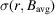with the diffraction-component precision index (DPI)  (p. 411) | html | pdf |
• Table 18.5.7.2. Examples of diffraction-component precision indices (DPIs)  (p. 411) | html | pdf |
• Table 18.5.7.3. Comparison of DPIs using R and R free   (p. 412) | html | pdf |
• Table 18.5.8.1.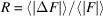as a function of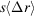in the Luzzati model for three-dimensional noncentrosymmetric structures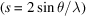(p. 413) | html | pdf |